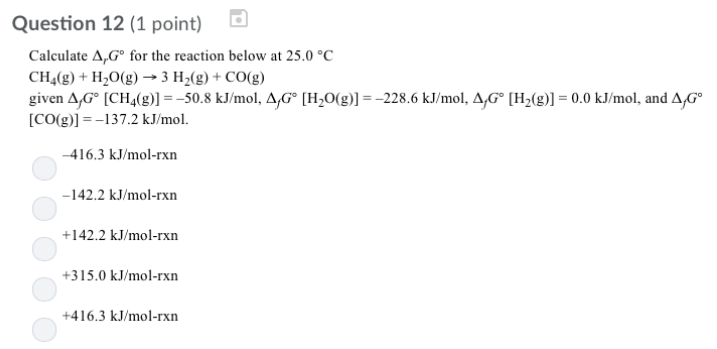# Problem: Calculate ΔrG° for the reaction below at 25.0°C CH4 (g) + H2O (g) → 3H2 (g) + CO (g)ΔfG° [CH4 (g)] = -50.8 kJ/mol, ΔfG° [H2O (g)] = -228.6 kJ/mol, ΔfG° [H2 (g)] = 0.0 kJ/mol, and ΔfG° [CO (g)] = -137.2 kJ/mol. (a) -416.3 kJ/mol-rxn (b) -142.2 kJ/mol-rxn (c) +142.2 kJ/mol-rxn (d) +315.0 kJ/mol-rxn (e) +416.3 kJ/mol-rxn

###### FREE Expert Solution
87% (448 ratings)###### Problem Details

Calculate ΔrG° for the reaction below at 25.0°C

CH4 (g) + H2O (g) → 3H2 (g) + CO (g)

ΔfG° [CH4 (g)] = -50.8 kJ/mol, ΔfG° [H2O (g)] = -228.6 kJ/mol, ΔfG° [H2 (g)] = 0.0 kJ/mol, and ΔfG° [CO (g)] = -137.2 kJ/mol.

(a) -416.3 kJ/mol-rxn

(b) -142.2 kJ/mol-rxn

(c) +142.2 kJ/mol-rxn

(d) +315.0 kJ/mol-rxn

(e) +416.3 kJ/mol-rxn